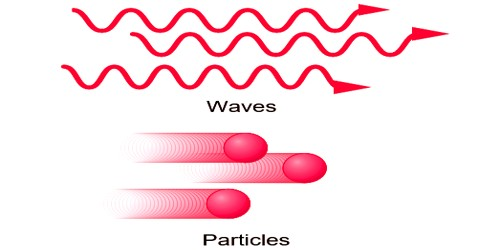# Difference between a Particle and a Wave

A particle occupies a well-defined position in space. It is localized. It is something that is localized in space and time. And exactingly speaking, this means its position can be narrowed down to greater and greater precision, but a table only exists on a length scale of a meter or so. Therefore a table isn’t exactingly a particle.

A wave is spread out in space. Two particles cannot be in a similar place at a similar time. A wave is just a function of position, which obeys a definite equation. What the function really represents could be whatever you’re trying to model: for example temperature, electric field, height against the horizontal position of a string, a real wave on the sea.

The concept of a particle and a wave can be understood by the different points of destination between then.

PARTICLE

1. A particle occupies a well-defined position in space i.g. a particle is localized in space e.g. a grain of sand, a cricket ball, etc.
2. When a particular space is occupied by one particle, the same space cannot be occupied simultaneously by any other particle. In other words, particles do not interfere.
3. When a number of particles are present in a given region of space, their total value is equal to their sum i.e it is neither less nor more.
4. The energy of a particle depends on its rest mass, potential energy, and kinetic energy. A particle is something that exists at only one point in space.
5. A particle is a small localized object to which can be ascribed several physical or chemical properties such as volume, density or mass.

WAVE

1. A wave is spread out in space e.g. on throwing a stone in a pond of water, the waves start moving out in the form of concentric circles. Similarly, the sound of the speaker reaches everybody in the audience. Thus a wave is delocalized in space.
2. Two or more waves can coexist in the same region of space and fence interfere.
3. When a number of waves are present in a given region of space, due to interference, the resultant wave can be larger or smaller than the individual waves i.e. interference may be constructive or destructive.
4. The energy of a wave depends on its frequency and amplitude. A wave has varying levels of intensity at different points in space and time.
5. A wave is a disturbance that transfers energy through matter or space, with little or no associated mass transport.

A fundamental difference between wave and particle nature of light as a particle it can explain the photoelectric effect and as a wave, it can explain the reflection, diffraction, etc. Wave is the disturbance of some quantity in space or intermediate while a particle has a definite mass concentrated on a small area. Wave does not have a dimension of size, only its wavelength and velocity can be calculated.

Properties of particles:

• A particle is a localized point in the space.
• More than one particle cannot be present at a given position in space.
• Particles do not show the phenomenon of interference.

Properties of waves:

• A wave is delocalized in space.
• Two or more than two waves can simultaneously exist at a given region of space.
• Waves show the phenomenon of interference.